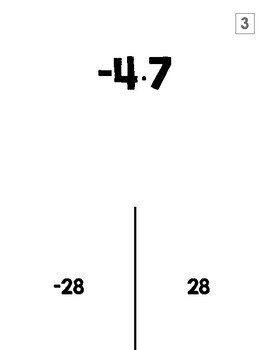digital

# Integer Operations Halloween Activity (TRICK or TREAT)Subject
Resource Type
Formats

TpT Digital Activity

PDF (435 KB|20 pages)
Standards
\$3.00
TpT Digital Activity
Add notes & annotations through an interactive layer and assign to students via Google Classroom.
\$3.00
TpT Digital Activity
Add notes & annotations through an interactive layer and assign to students via Google Classroom.

### Description

A fun way to practice integer operations! Students answer a question with 2 answer choices and will lift the flap to see if it is a "trick" (incorrect answer) or "treat" (correct answer). There are 16 questions in which students add, subtract, multiply, or divide positive and negative numbers. Students love this! (Especially if real treats are involved). I hope you love it and your students learn it!

You may also like...

Adding and Subtracting Integers Halloween Color Page

Fall Integer Operations Scavenger Hunt

HALLOWEEN Two Step Equations Candy Corn Activity

Total Pages
20 pages
Included
Teaching Duration
30 minutes
Report this Resource to TpT
Reported resources will be reviewed by our team. Report this resource to let us know if this resource violates TpT’s content guidelines.

### Standards

to see state-specific standards (only available in the US).
Understand that integers can be divided, provided that the divisor is not zero, and every quotient of integers (with non-zero divisor) is a rational number. If 𝘱 and 𝘲 are integers, then –(𝘱/𝘲) = (–𝘱)/𝘲 = 𝘱/(–𝘲). Interpret quotients of rational numbers by describing real-world contexts.
Understand that multiplication is extended from fractions to rational numbers by requiring that operations continue to satisfy the properties of operations, particularly the distributive property, leading to products such as (–1)(–1) = 1 and the rules for multiplying signed numbers. Interpret products of rational numbers by describing real-world contexts.
Understand subtraction of rational numbers as adding the additive inverse, 𝘱 – 𝘲 = 𝘱 + (–𝘲). Show that the distance between two rational numbers on the number line is the absolute value of their difference, and apply this principle in real-world contexts.
Apply and extend previous understandings of addition and subtraction to add and subtract rational numbers; represent addition and subtraction on a horizontal or vertical number line diagram.